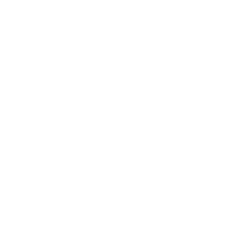## turnRight(angle) 向右转

`turnRight()` 是一个可以修改 turtle 当前面向方向的方法之一。通过跟类似 moveForward() 的方法配合使用，你可以移动到屏幕的任何位置绘制图形。

### 示例代码

``````
// 让 turtle 向右转 90 度（默认）。
turnRight();
``````

``````
// 向右转，向左转。
moveForward();
turnRight();
moveForward();
turnLeft();
``````

``````
// 只向右转画出字母 W
turnRight(150);
moveForward();
turnRight(-120);
moveForward();
turnRight(120);
moveForward();
turnRight(-120);
moveForward();
``````

``````
// 画 25 角星？画之前好好算下角度。
var points = 25;
var exteriorAngle = 180.0 - (180.0 / points);
for (var i = 0; i &lt; points; i++) {
moveForward(200);
turnRight(exteriorAngle);
}
``````### 语法规则

``````
turnRight(angle);
``````

### 参数说明

angle number No 指定转动的角度（默认为 90 度）。

### 提示说明

• 在执行向右转方法时，可以指定一个负的角度（小于零）。实际会变成向左转。
• 有三种方法可以让 turtle 原地改变方向：
• turnRight(angle) 将 turtle 在当前方向基础上向右转指定的 `angle` 角度。turtle 位置保持不变。
• turnLeft(angle) 将 turtle 在当前方向基础上向左转指定的 `angle` 角度。turtle 位置保持不变。
• turnTo(angle) 将 turtle 当前方向设为指定的 `angle` 角度。turtle 位置保持不变。0 度为垂直向上，90 度为水平向右，180 度为垂直向下，270 度为水平向左。

• UI Controls
• Canvas
• Data
• Turtle
• Control
• Math
• Variables
• Functions